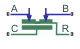# Cylinder Friction (IL)

Adds friction to cylinders with moving components

• Library:
• Simscape / Fluids / Isothermal Liquid / Actuators / Auxiliary Components

•## Description

The Cylinder Friction (IL) block models friction on a moving cylinder. The total friction is a combination of Stribeck, Coulomb, and viscous effects. The Coulomb friction component includes any initial force applied by the seal and the influence of pressure in the cylinder.

The Stribeck and viscous forces are calculated in the same way as for the Simscape Foundational Library Translational Friction block.

### The Stribeck Friction and Viscous Friction Forces

The Stribeck friction force is the dominating friction component at low velocities. It is calculated as:

`${F}_{Stribeck}=\sqrt{2e}\left({R}_{break,Coulomb}-1\right)\cdot {F}_{C}\left(\frac{\upsilon }{{\upsilon }_{static,th}}\right){e}^{-{\left(\frac{\upsilon }{{\upsilon }_{static,th}}\right)}^{2}},$`

where:

• Rbreak,Coulomb is the ratio of the breakaway force to the Coulomb force: ${R}_{Break,Coulomb}=\frac{{F}_{break}}{{F}_{Coulomb}}.$

• ν is the velocity of the cylinder, $\upsilon ={\upsilon }_{R}-{\upsilon }_{C}$.

• νstatic,th is the threshold velocity for static torque:

`${\upsilon }_{static,th}=\sqrt{2}{v}_{break},$`

where vbreak is the Breakaway friction velocity. A transition range is established between 0 and the threshold velocity for static torque to ensure smooth modeling of the friction force.

• FC is introduced in The Coulomb Friction Force section below.

The viscous friction force is based on the Viscous friction coefficient of the working fluid, fviscous, and is proportional to the cylinder velocity:

`${F}_{viscous}={f}_{viscous}\upsilon .$`

### The Coulomb Friction Force

The Coulomb friction force is a force that acts normal to the friction surface. The cylinder motion creates a radial stress inside the fixed cylinder casing, which increases as the cylinder compresses the internal fluid. The radial stress is normal to the cylinder motion, and results in a Coulomb friction force that opposes cylinder motion.

The Coulomb frictional force is calculated as:

`${F}_{Coulomb}={F}_{c}\mathrm{tanh}\left(\frac{\upsilon }{{\upsilon }_{Coulomb,th}}\right),$`

where ν is the relative velocity between ports R and C (νRνC), and νCoulomb,th is the threshold velocity for Coulomb force:

`${\upsilon }_{Coulomb,th}=\frac{{v}_{break}}{10}.$`

Note that the threshold velocity for Coulomb force is a different quantity than the threshold velocity for the static force, which is used to calculate the Stribeck friction force, although they both act as a threshold region to ensure smooth modeling of the Coulomb and Stribeck forces.

FC is the force contribution from seal preloading (the Preload force) and the pressure in the cylinder:

`${F}_{C}={F}_{preload}+{f}_{Coulomb}\left({P}_{A}+{P}_{B}\right),$`

where fCoulomb is the Coulomb friction force coefficient. PA and PB, the pressures at cylinder ports A and B, are gauge pressures with respect to the environmental pressure, specified either as atmospheric or another user-defied value in the Environment pressure specification parameter.

## Ports

### Conserving

expand all

Liquid entry or exit point to the orifice.

Liquid entry or exit point to the orifice.

Mechanical port for cylinder housing motion, in m/s.

Mechanical port for cylinder rod motion, in m/s.

## Parameters

expand all

Ratio of the breakaway force to the Coulomb friction force.

Threshold for movement against friction force.

Initial force in the cylinder due to seal assembly.

Coulomb friction force coefficient.

Viscous friction coefficient. This parameter is used to calculate the viscous friction force.

Identifies pressure reference for the Coulomb friction calculation.

User-defined pressure reference for the Coulomb friction calculation.

#### Dependencies

To enable this parameter, set Environment pressure specification to ```Specified pressure```.

## Version History

Introduced in R2020a